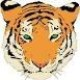2 check digits for a EAN13 barcode FollowPascal vd linden

Hello,

i am supposed to make an EAN13 barcode with a 6 digit article nr. + a 5 digit weight.

And they want 2 check digits, 1 before the weight and 1 after

this is an example of my barcode:

282030 CD 103504 (the last digit '4' is the automatic generated CD)

282030 (art.nr.) cd 10,350 (weight) cd

is this possible ?Pete Thane

Yes it is possible to do this but the final check digit would not be a 4 (see below). Also the code would be completely out of spec for the symbology as it would no longer follow the standard for an EAN13 barcode, but if it is used internally that shouldn't be an issue.

You would need to make the code out of three separate substrings

1. The first would be the article number and  you would need to give the string a name (from the the Change Data Source Name button, such as ArtNo)
2. Substrting two would need to be a Visual basic Script field. If the ArtNo field is fixed then you should make it a Multi-Line Script but if it comes from a Database or a manual input on a Data Entry then this would need to be Event Controlled Script using either the OnNewRecord or OnPostPrompt option.  The process to calculate the check digit can be found in the right hand column in the scripting screens in the Functions>Check Digit option and, assuming you are using the usual EAN13 check digit calculation then the UCCMod10 function is the one you need replacing the highlighted String by double clicking on the name you gave to the 1st substing. A sample of the VB can be seen below
3. The final substring would be the weight and BarTender would automatically add on the standard CD at the end of these 3 strings.

Value = UccMod10(Format.NamedSubStrings("ArtNo").Value)

The final barcode number would be

282030 9 10350 3Pascal vd linden

Thank you for the reply !

I created 3 strings and did exactly what you said.

it works half way

282030 9 10350 3 this is my barcode now

the art.no. is fixed but the weight comes from a database.

my client said that the control digit what is a 9 in the example is wrong.

i printed a number of labels and the control digit stays the same, its Always a 9

is there a way to fix this ?Pete Thane

The 9 check digit is based on the article number using the UCCMod10 format and so if the Article Number is always the same then it will always be a 9.

How do they want the check digit before the weight to be calculated?Pascal vd linden

they want the check digit before the weight and after the weight.

I Really don´t know how, i have a labelsystem for pieces and this system gives me the following option:

FF CCCCC PCD WWWWW CD

and with that barcode lay-out i get the right check digits.

i've printend 4 different weights and these are my results:

2820306010453

2820300006605

2820304009459

2820301007601

282030 is my Art.no.

1.045,  0.660,   0.945,  0.760 are my weights

i'm guessing this isn't possible in bartender ?Pete Thane

Yes it is possible, as long as you know the formula used to calculate the central check digit.

The check digits 3,5,9,1 in the samples above are the standard EAN CD and are as result of formula based on the previous 12 characters to give a check character in UCCMod10 format. The number after the Art no does not seem to confirm to this.